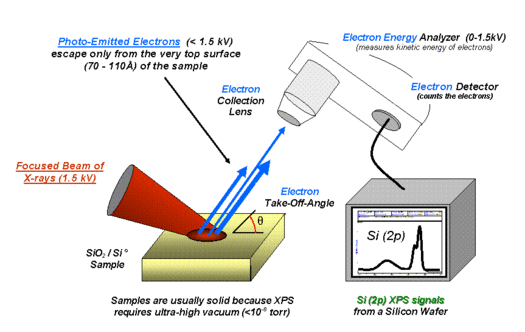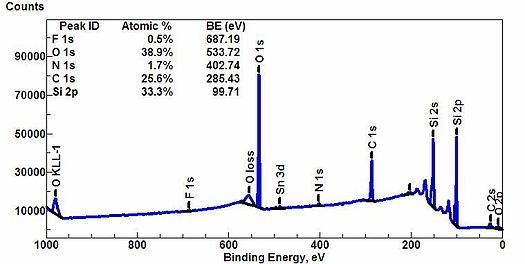# X-ray Photoelectron Spectroscopy (XPS)

Student authors: Crystal Good 2018 & Horace Facey 2019

## How XPS works

X-rays (photons) are shot onto a sample, and when electrons in the sample absorb enough energy, they are ejected from the sample with a certain kinetic energy. The energy of those ejected electrons is analyzed by a detector and a plot of these energies and relative numbers of electrons is produced. Electrons of different energies follow different paths through the detector which allows the computer to differentiate the electrons and produce the spectra seen below.Figure $$\PageIndex{1}$$: Diagram depicting the instrumentation of the X-ray photoelectron spectrometer.

(Image released to public domain. Source: https://en.wikipedia.org/wiki/X-ray_...n_spectroscopy)

Atoms present in compound being tested by XPS are determined according to the equation:Here, binding energy is the energy of an electron attracted to a nucleus; photon energy is the energy of X-ray photons being used by the spectrometer, and the kinetic energy is the energy of the ejected electrons from the sample. The work function is a correction factor for the instrument and correlates to the minimum energy required to eject an electron from an atom (see the photoelectric effect for more info, but not necessary to understand this). The work function and photon energy are known and the kinetic energy is measured by the detector. That leaves the binding energy as the only unknown, which can then be determined. As electrons are in orbitals farther from the nucleus, less energy is required to eject them, so the binding energy is lower for higher orbitals. Electrons contained in different subshells (s,p,d, etc.) have different energies as well. By showing the energy of electrons emitted from a material, XPS allows for the composition of a material to be determined.

Additionally, chemical shifts can be determined using XPS. This is owing to the fact that binding energy doesn’t only depend on the shell of the electron. It also depends on the environment, that is, the bonds that the atom in question partake in. Therefore, a primary carbon would have a slightly different binding energy than a carboxyl carbon, for example.Figure $$\PageIndex{2}$$: XPS spectra. Graph showing the binding energies of electrons from different orbitals (F1s, O1s, Si2p, etc.) and their intensities which tell the atomic composition of the sample based on the amounts of each electron from different orbitals present. (Figure courtesy of the creative commons license. Source: https://en.wikipedia.org/wiki/X-ray_photoelectron_spectroscopy).

## How to interpret the data it generates

1. Peaks from the XPS spectra give relative number of electrons with a specific binding energy. The shorter the peak, the less electrons represented. For example, if a peak, A, is half the height of another peak B, that means there were half as many electrons detected with the binding energy at A compared to the number of electrons detected with the binding energy at B. Therefore, the peak intensities give information about the percent composition of a material. As seen in the figure above, the O1s has the largest peak, and it shows that the atomic composition of oxygen is the greatest.

2. The greater the binding energy, the greater the attraction of that electron to the nucleus. i.e. peaks from electrons in 1s will have a greater energy than peaks representing electrons from 2s. Electrons in 2s will have greater energy than those in 2p. Some instruments have peak identification features, but otherwise, the identification of peaks/lines on the spectra can be completed by looking at standards of different materials. Moulder (1992) shows examples of these standards to help interpret the spectra.

## Error/difficulties in reading the spectra

1. Be careful about the direction of the scale for binding energy. There are examples figures having zero is on the left and some having it on the right.
2. Some lines/peaks may overlap, making it difficult to determine if the electrons represented are coming from different atoms
3. Auger lines: lines with complex patterns, not related to the kinetic energy from ionization of electrons. It is important to identify these lines first.
4. X-ray ghost lines: small lines or peaks that are shifted a little more than expected due to additional x-ray sources than from the instrument
5. Only the surface is tested, so the behavior at the surface and bulk may be quite different.

## Good literature examples

Penke, Y.K.; Anantharaman, G.; Ramkumar, J.; Kamal, K. “Aluminum Substituted Cobalt Ferrite (Co−Al−Fe) Nano Adsorbent for Arsenic Adsorption in Aqueous Systems and Detailed Redox Behavior Study with XPS.” ACS Appl. Mater. Interfaces. 2017, 9, 11587−11598.

This paper discusses the adsorption of arsenic into aluminum substituted cobalt ferrite. XPS is used to confirm the presence of Al, and then the instrument confirms the oxidation or reduction of arsenic when it is adsorbed into the material.